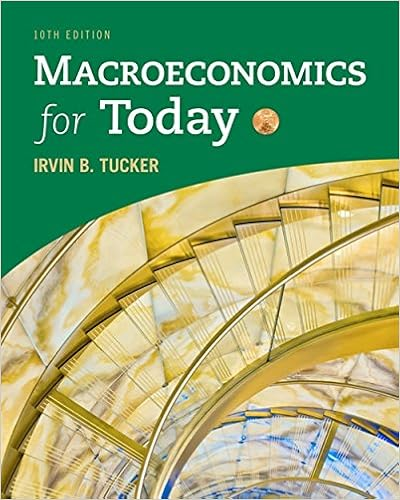The above figure represents the cost curves for a

• Test Prep
• 6
• 98% (259) 253 out of 259 people found this document helpful

This preview shows page 3 - 5 out of 6 pages.

We have textbook solutions for you!
The document you are viewing contains questions related to this textbook.The document you are viewing contains questions related to this textbook.
Chapter 1A / Exercise 20
Macroeconomics for Today
TuckerExpert Verified
6. The above figure represents the cost curves for a perfectly competitive firm. Above what price will the firm be making positive economic profits? A) \$5 B) \$12
We have textbook solutions for you!
The document you are viewing contains questions related to this textbook.The document you are viewing contains questions related to this textbook.
Chapter 1A / Exercise 20
Macroeconomics for Today
TuckerExpert Verified
C) \$4 D) \$2 Feedback: At \$5, the firm is making zero economic profits because at that price, P=ATC at the profit maximizing output. Therefore, above \$5, the firm will be making positive economic profits. Table for Individual Question Feedback Points Earned: 0.0/1.0 Correct Answer(s): A
7. In the above figure, the left hand side graph represents a perfectly competitive industry and the right hand side graph represents a perfectly competitive firm. Suppose that the original demand curve is D 0 , as labeled. Due to an increase in income, the demand curve shifts to the right. Then,
Table for Individual Question Feedback Points Earned: 1.0/1.0 Correct Answer(s): A
•••